# Electronics and Communication Engineering - Exam Questions Papers

16.

Consider a parallel plate capacitor being charged. Identify the incorrect statements

 A. Energy stored in the capacitor does not enter it through the connecting wires but through the space around the wires and plates uf capacitor B. Rate at which energy flows into this volume is equal to the integration of the Poynting vector over the boundary of the volume between the plates. C. The Poynting vector points everywhere radially outward of the volume between the plates D. The Poynting vector points everywhere radially into the volume between the plates.

Explanation:

In a parallel plate capacitor, current passes through the connectors.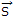is the Poynting vector which points radially into the volume.

17.

Find equivalent resistance. Each resistance is l Ω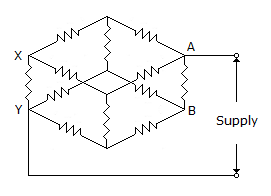A. 5/12 Ω B. 5/6 Ω C. 6/5 Ω D. 6/10 Ω

Explanation:

Cut the cuboid at XY - AB plane. This will make two parallel and symmetrical loops. Resistance between XY and AB = 1 Ω Splitting it in two equal values resistances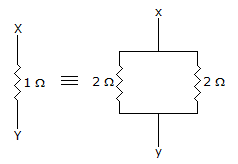Solving one of the loop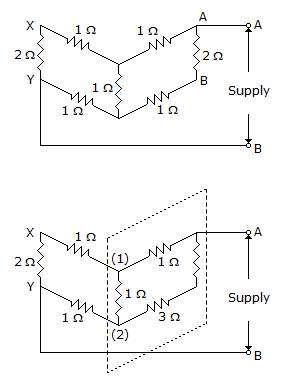Use Δ - Y transformation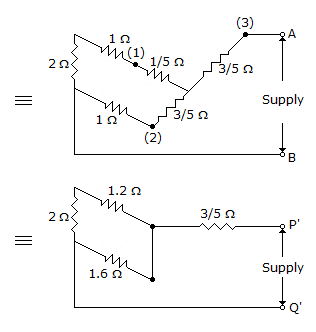⇒ RP'Q' =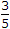+ (1.6 || [1.2 + 2]) = 1.667 Ω

Req = RP'Q' || RP'Q' =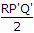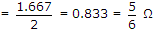.

18.

The following circuit is implementation of:

1. sum of full adder
2. carry of half adder
3. difference of full subtractor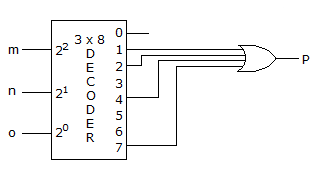A. 1 only B. 3 only C. 2 only D. 1 and 2 both

Explanation:

Let the two bits be A and B. Then the truth table be: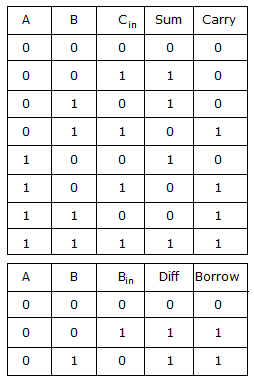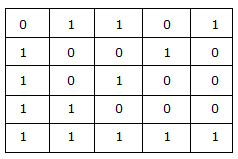Now it is evident from the truth tables that Sum and Difference are same.

19.

Find ZTH, VAB current through 10 Ω resistance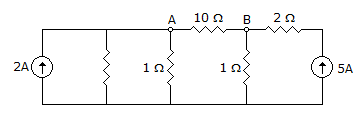A.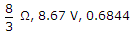B.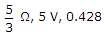C.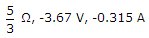D.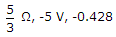Explanation: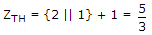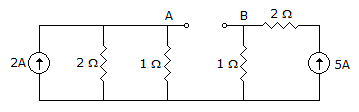Apply KCL at node A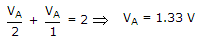VB = 5 x 15 V

VAB = VA - VB = 1.33 - 5 = - 3.67 V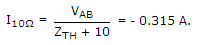20.

The region between a pin of parallel perfectly conducting planes of infinite extent is y and z directions is partially filled with a dielectric as shown below.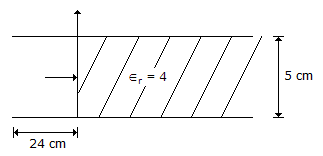A 30 GHz TE10 wave is incident on the air-dielectric interface. The VSWR at the interface is

 A. - 2 B. 2 C. 0 D. 1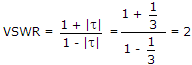.